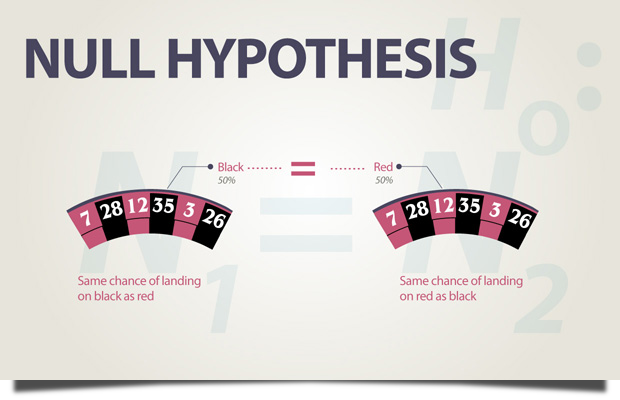# Null Hypothesis

Null Hypothesis is a statement about parameters being tested in a significance test that is a statement of no effect, no difference.

- Triola (2009).

# ExampleThis example shows the statement/assumption that the probability of landing on the black square has the same probability of landing on the red square. This is just one example of a null hypothesis.

## Related Terms

Alternative Hypothesis

Hypothesis